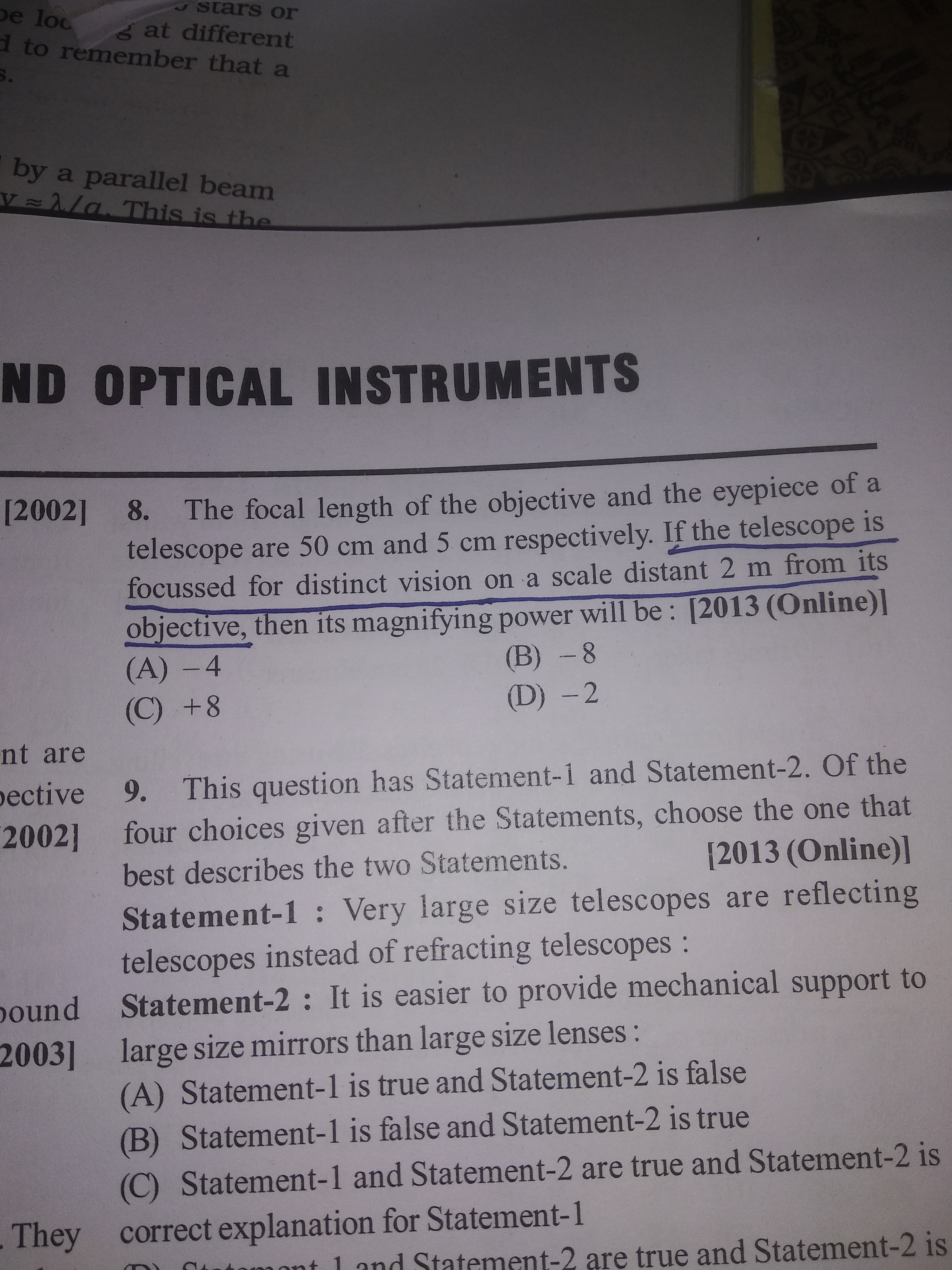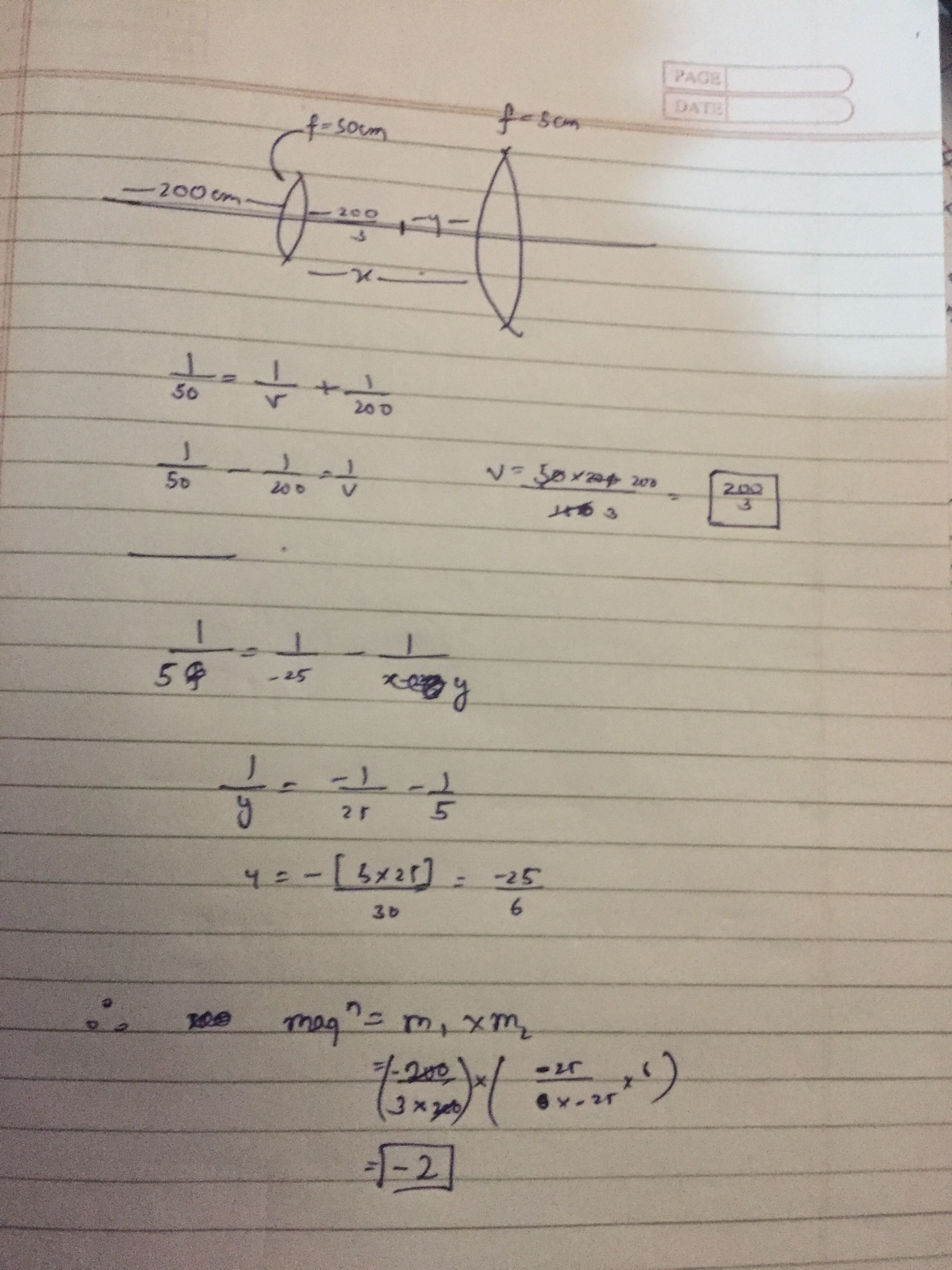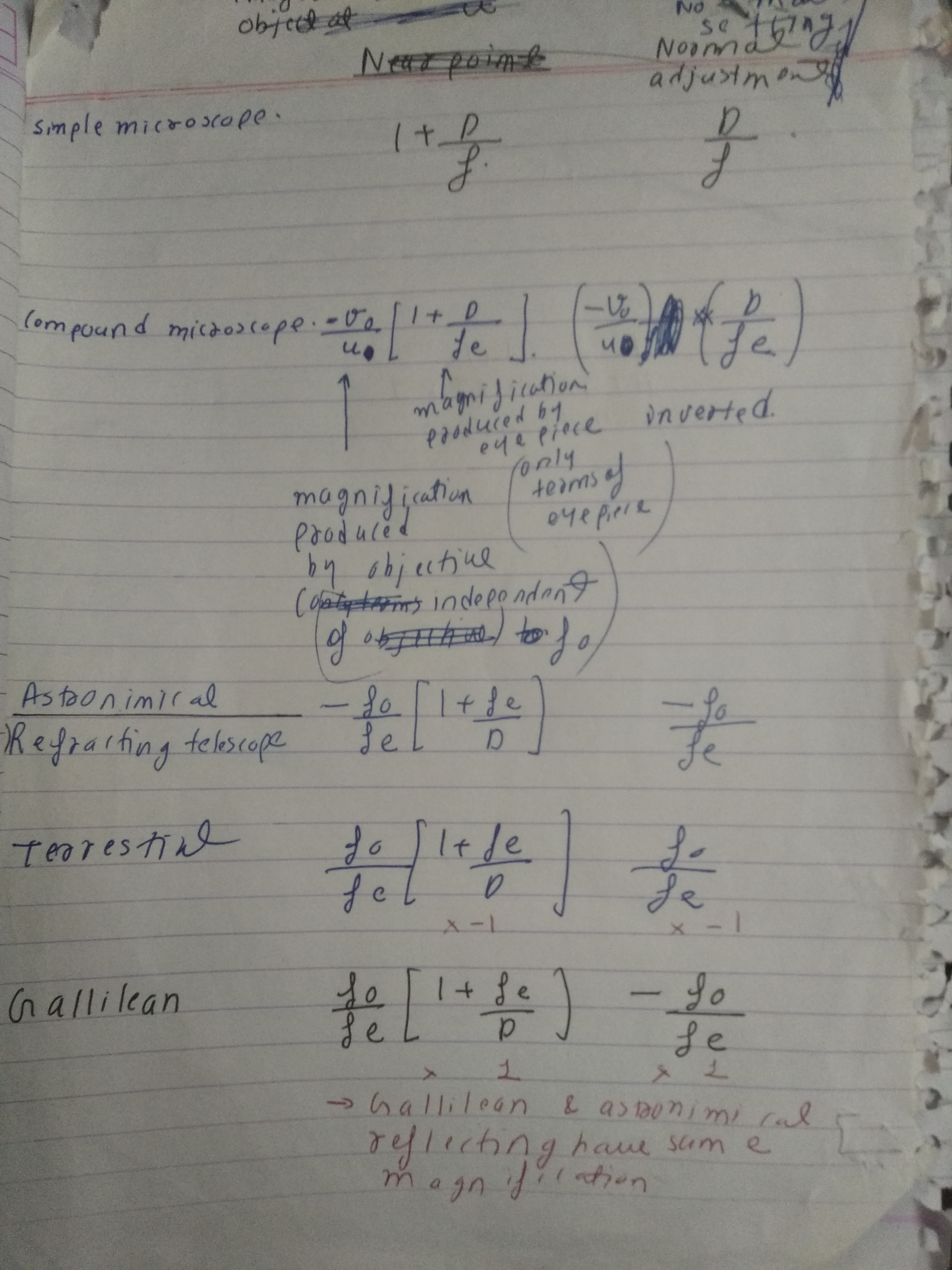# Doubt from telescope magnificationQ8
Why can't we simply use formula here? What is the language of question telling us?

Humans can see objects clearly only if it is at a distance of 25cm or greater and hence the image must be formed at 25 cm for distinct vision

Can you solve it completely?1 Like

@Hritik_2020 is it clear to you now ?

Why we cant do this question by direct formulae of magnification of telescope?

Which formula ?These ones

You can use these also here use the formula of compound microscope with image at D i.e 1st formula of compound microscope, you’ll get the same answer

Isn't it is a telescope?

No it isn’t a telescope, telescope is used to see very distant objects , here the objects are at finite distance (2m)

Was there something in the question which made you think that it’s a telescope ?

I understood why formulae of microscope is used but it was given in question it is a telescope that's why

1 Like

Yeah ! 2m object sounds quite distant to use for a microscope so they mist have mentioned telescope bit nevertheless it will function as a compound microscope only because the formula derived for telescope is for a very distant objects which thus form image at focus which isn’t true in this case

1 Like

Which part of question indicate that final image is formed at D?
As question says telescope is focused for distinct vision, doesnt it indicate the final image should be formed at infinity ?

It says “focused for distinct vision” i.e image must be at D i.e 25 cm not infinity

We can't use the normal formula because in normal formula we will have to put value of u' v' and all these values will require the distance between the lenses or separation between them.

@Shaquib u is given and v can be calculated without knowing the separation between the lenses .
We can apply the formula directly to get the answer

The 25cm you used it should be mention in question.

It is mentioned that image is formed for distinct vision i.e that 25 cm is given